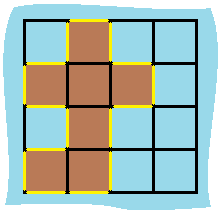# LeetCode：Island Perimeter - 岛屿周长

2017/09/26 11:15

1、题目名称

Island Perimeter（岛屿周长）

2、题目地址

https://leetcode.com/problems/island-perimeter/description/

3、题目内容

You are given a map in form of a two-dimensional integer grid where 1 represents land and 0 represents water. Grid cells are connected horizontally/vertically (not diagonally). The grid is completely surrounded by water, and there is exactly one island (i.e., one or more connected land cells). The island doesn't have "lakes" (water inside that isn't connected to the water around the island). One cell is a square with side length 1. The grid is rectangular, width and height don't exceed 100. Determine the perimeter of the island.

Example:

[[0,1,0,0],
[1,1,1,0],
[0,1,0,0],
[1,1,0,0]]

Explanation: The perimeter is the 16 yellow stripes in the image below:4、解题方法

/**
* LeetCode 463 - Island Perimeter
* @文件名称 Solution.java
* @文件作者 Tsybius2014
* @创建时间 2017年9月26日10:30:40
*/
class Solution {

/**
* 计算岛屿周长
* @param grid
* @return
*/
public int islandPerimeter(int[][] grid) {

if (grid.length == 0 || grid.length == 0) {
return 0;
}

int perimeter = 0;
for (int i = 0; i < grid.length; i++) {
for (int j = 0; j < grid[i].length; j++) {
if (grid[i][j] == 1) {
if (i == 0 || grid[i - 1][j] == 0) {
perimeter++;
}
if (i == grid.length - 1 || grid[i + 1][j] == 0) {
perimeter++;
}
if (j == 0 || grid[i][j - 1] == 0) {
perimeter++;
}
if (j == grid.length - 1 || grid[i][j + 1] == 0) {
perimeter++;
}
}
}
}

return perimeter;
}
}


END

0
0 收藏

### 作者的其它热门文章0 评论
0 收藏
0